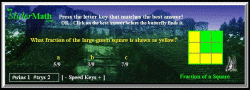< WIDTH="260" ALIGN="left">Teaching & Testing  Help for Math  View Page 1 : Arithmetic+Integers+Exponents+Fractions

Sequenced Math Help Games
SliderMath.com Games. For juniors & seniors. For computers, cell phones & tablets.
Practice your Math from the convenience of your home when necessary.Algebra Teaching Help - Polynomials & Factoring: Add & Subtract Like Terms  Add 3 like Terms  Add 3 Terms mixed  Add 3 Terms variables  Add 4 Terms  Add & Subtract 4 Terms Polynomial Types :  Monomials, Binomials, Trinomials  Multiply Monomial x Binomial  Monomial x Trinomial  Divide Monomials & Binomials by a Monomial  Divide Binomials & Trinomials by a Monomial  Polynomial Word Expressions  Simplify Polynomials  Binomial x Binomial  Binomial x Trinomial Divide a Polynomial by a Binomial Factoring:   Factor Numbers   Common Factor 2 Terms   Common Factor Trinomials Difference of Squares   Perfect Trinomial Squares   Factor Trinomials   Complete a Square Graphing Math Help:  Formulas & Data    Data Pairs    Relations & Ordered Pairs Graph Co-ordinates:  Point as Ordered Pair  Positive & Negative Pairs    Slope as  Rate of Change  Slope from 2 Points  Speed & Slope  Relations & Rates  Rates & Ordered Pairs     Points on  y=ax & y=ax2     Slope & Y-intercept  y=mx+b     Line Slope-Point Form     Name parabolas  y=ax2+b  Parabola Vertex    Circles   Power Functions   Exponentials   Tangents & Speed Fun Equations Teaching Help: Solve Equations:  4 Methods (+-x/) using 2 Steps Equation Types ay+b=c, ay-b=c   ay+b=cy+d, ay-b=cy+d   Equation Word Problems Trigonometry Teaching Help: Slope Tangent Ratio   Tangent Function   Sine Function   Cosine Function   TanSineCos   CotanCosecSec Trig Special Angles   Trig Quadrant Angles   Primary Trig Word Problems Unit Circle & Sine Wave   Sine Wave Graphs Calculus Derivative Rules Help: Powers & Constants    Derivatives of Roots    Derivative of a Sum Derivative Product Rule    Products with Sine & Cosine    Chain Rule